### Digital Logic IC Fundamentals: Brief Review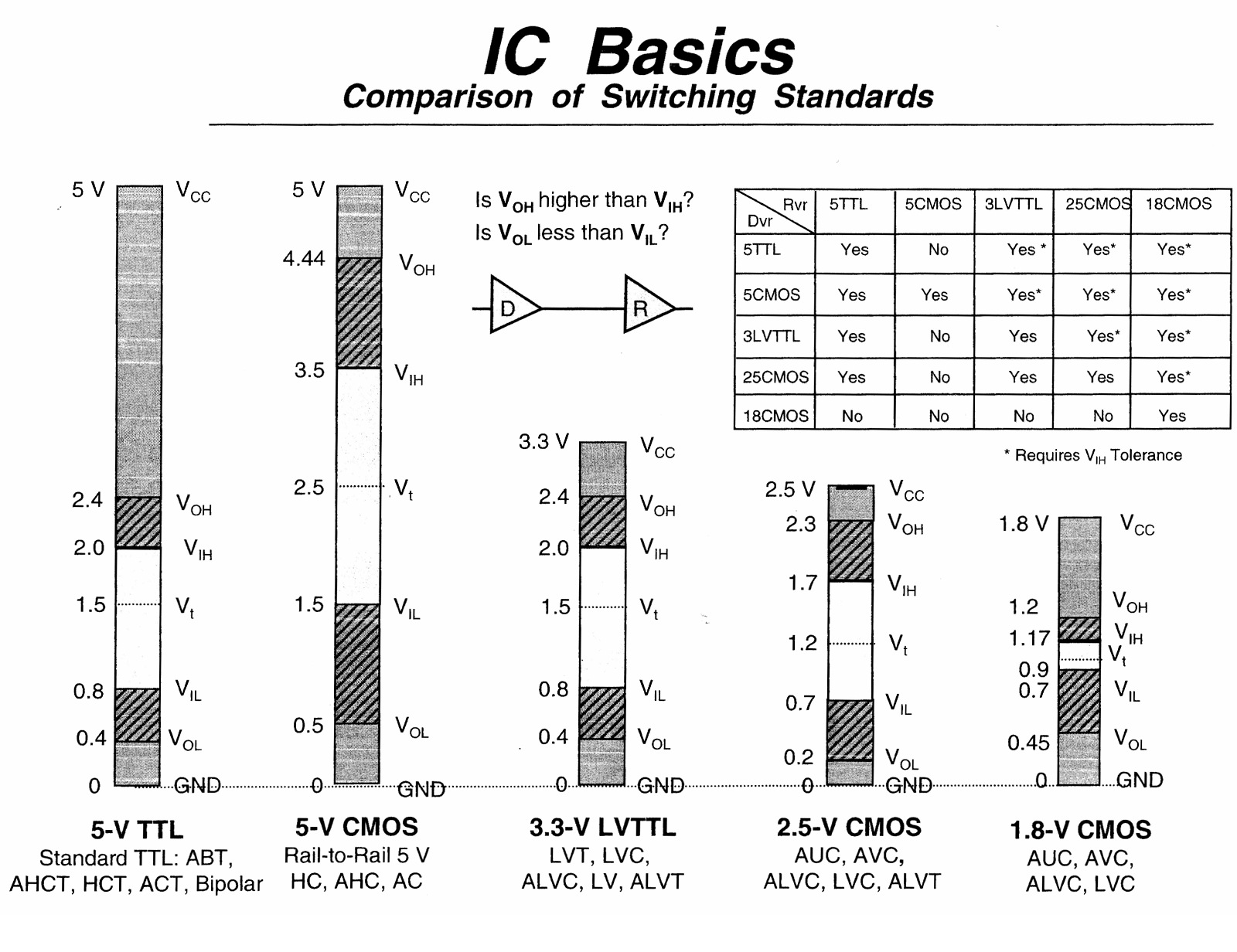Two mainstream Logic Families:
1. TTL
2. CMOS
To know more about the Logic Families go through Texas Instrument's documentation "Logic Families" on their website www.ti.com/ (which I think is one of the biggest and oldest resources for semiconductor-related information) OR click here to view the document directly.

De Morgan's Law when applied to Engineering:

• Conjunction = AND Gate
• Disjunction = OR Gate

Bubble Pushing in De-Morgan's Law explained below: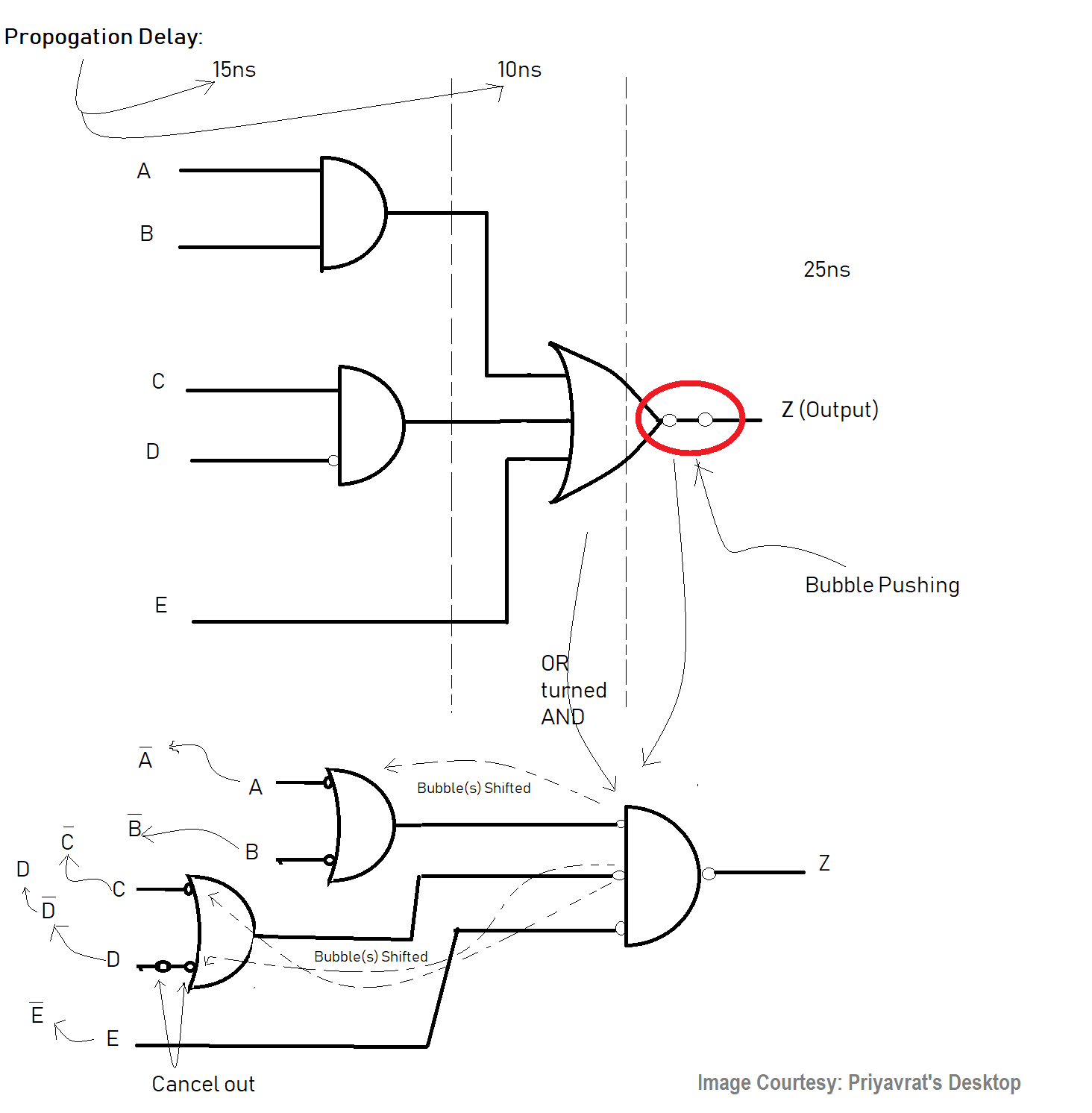A datasheet has the following information in some order:
1. A picture of the component and its pinout.
2. Images of various package options.
3. A short description of the component.
4. Ordering information and coding part numbers.
5. Functional descriptions.
6. Application notes and examples.
7. AC and DC characteristics.
8. Mechanical drawings and footprints.
9. Distribution, sales, and technical support contacts.

Interpreting TTL Datasheets:
1. VCC (Supply voltage) – This is the min and max for the main supply. Usually set to +5V.
2. VOH (High-level output voltage) – When outputting a logic HIGH “1”, it’s guaranteed to be greater than or equal to this value.
3. VOL (Low-level output voltage) - When the outputting a logic LOW “0”, it’s guaranteed to be less than or equal this value.
4. VIH (High-level input voltage) – This is the minimum voltage input to represent a logic HIGH “1”.
5. VIL (Low-level input voltage) - This is the maximum voltage input that represents a logic LOW “0”.
6. IOL (Low-level output current) – This is the amount of current the device can sink when driving a logic LOW “0” output.
7. IOH (High-level output current) - This is the amount of current the device can source when driving a logic HIGH “1” output.
8. IIL (Low-level input current) – This is the amount of current necessary to drive an input to logic LOW “0”.
9. IIH (High-level input current) - This is the amount of current necessary to drive an input to logic HIGH “1”.
10. TPHL (Propagation delay from input to output for a high to low transition) – Assuming that an input is currently being driven at a logic HIGH “1” at time t=0, then the input switches instantaneously to logic LOW “0”, there will be a finite amount of time this signal takes to propagate thru the device, this propagation delay (usually in nanoseconds) is TPHL.
11. TPLH (Propagation delay from input to output for a low to high transition) - Assuming that an input is currently being driven at a logic LOW “0” at time t=0, then the input switches instantaneously to logic HIGH “1”, there will be a finite amount of time this signal takes to propagate thru the device, this propagation delay (usually in nanoseconds) is TPLH.
12. FMAX (Maximum switching frequency) – For clocked synchronous devices, this is the fastest the device can be clocked at and be reliable. Flip flops, counters, shift registers, etc. would be examples of chips that have an FMAX rating.
13. TA (Temperature ambient) – The range

Interpreting CMOS Datasheets:

CMOS differs from TTL is that CMOS circuit are made from field effect transistors rather than
bipolar transistors, CMOS is very low power, but has high capacitance loading.

Thus, many of the datasheet elements are similar, but there are a few different elements relevant for CMOS only:
1. VCC (Supply voltage) – This is the min and max for the main supply. Usually set to +5V.
2. FANOUT – Since CMOS chips are used to replace TTL chips now, a standard arose where the number of TTL inputs a CMOS output could drive was used to measure the CMOS chip’s output ability. So most CMOS outputs have a “fanout” of 8-20, meaning a single CMOS output can drive 8-20 TTL inputs. Of course, the more you “load” any output CMOS or TTL, the more degraded the signal will get, so I like to use 50% loading at most and play it safe.
3. VOH (High-level output voltage) – When outputting a logic HIGH “1”, it’s guaranteed to be greater than or equal to this value.
4. VOL (Low-level output voltage) - When the outputting a logic LOW “0”, it’s guaranteed to be less than or equal this value.
5. VIH (High-level input voltage) – This is the minimum voltage input to represent a logic HIGH “1”.
6. VIL (Low-level input voltage) - This is the maximum voltage input that represents a logic LOW “0”.
7. II (Input leakage current) – The amount of current CMOS input sources or sinks due to “leakage”. Usually negligible, on the order of 1uA.
8. CI (Input capacitance) – This is the amount of capacitance a CMOS input has on the order of 1- 10 pF usually. This adds up fast though and can slow your signal transition times down due to RC charging.
9. CL (Load Capacitance) – This is the amount of capacitance a given CMOS output can drive.
10. TPHL (Propagation delay from input to output for a high to low transition) – Assuming that an input is currently being driven at a logic HIGH “1” at time t=0, then the input switches instantaneously to logic LOW “0”, there will be a finite amount of time this signal takes to propagate thru the device, this propagation delay (usually in nanoseconds) is TPHL.
11. TPLH (Propagation delay from input to output for a low to high transition) - Assuming that an input is currently being driven at a logic LOW “0” at time t=0, then the input switches instantaneously to logic HIGH “1”, there will be a finite amount of time this signal takes to propagate thru the device, this propagation delay (usually in nanoseconds) is TPLH.
12. FMAX (Maximum switching frequency) – For clocked synchronous devices, this is the fastest the device can be clocked at and be reliable. Flip flops, counters, shift registers, etc. would be examples of chips that have an FMAX rating.
13. TA (Temperature ambient) – The range of ambient air temperatures that the device can operate at without external heat management.

Example Inverter Digital IC - SN74HC04 (datasheet, Source: TI [Texas Instruments]):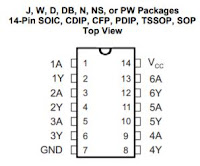Inverter(s) pin connections. -- *Connect in the same direction.TTL Output *Current flow shown in the diagram.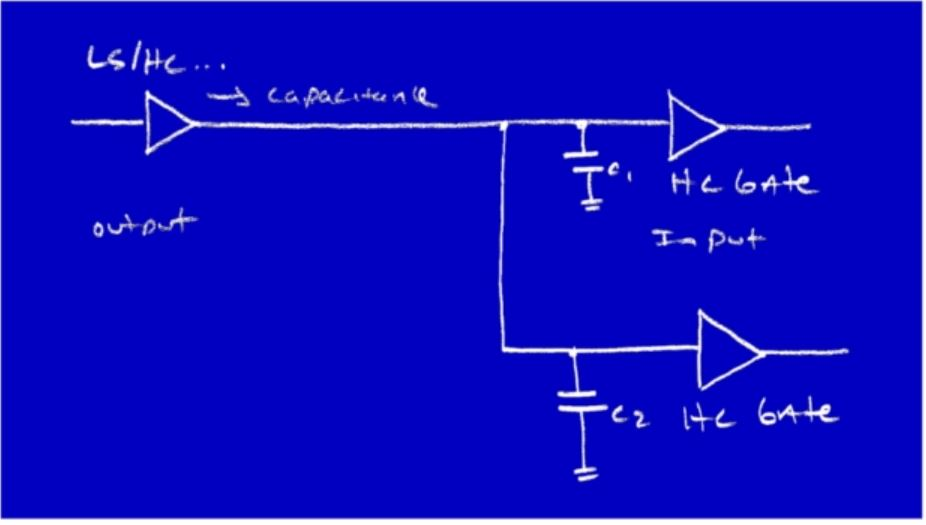HC Gates act like Capacitors Loading the Output
• See the Output is on the left side. Now, each of the HC Gates looks like a Capacitor to this Output. So it can drive only that many gates. So, we need to make sure we connect only to that many gates otherwise we won't be able to drive it.
• Thus, CL = how much total capacitance we can drive and CI = input capacitance (that is how the gates look like on the right side in the above diagram as far as input capacitance).
• So, when you hook these things together, you have to have enough drive to drive all of these gates. *Remember, the capacitor takes time to charge-up and you are going to violate the timings of this/these IC(s) and the datasheet specs.
• The IC would still work but it will not work as per the datasheet and you won't be able to maintain the spee
• When you mix TTL and CMOS, you need to be careful because in one case you need current and in the other case you need voltage and also, the voltages are slightly different.

Tri-State Logic:When Enable is on, this acts like a buffer.

Example using Enable (/En): Data Stream Selector with Tri-State Gates• For example, we have two TTL RS-232 Signals, both coming from a different computer. So, if you want to select which should be used to produce the output, hence the following must occur:
• Tie them together. The problem is, when this serial-data came in here it would cause shorting. So, we wire them together and not just the gate outputs. But, when we have this Output Enable (these tri-state gates on them), both are connected to the line with a switch. Thus, the switch selects which one should we enable and we are not turning on/off the power supply.

*The most common through-hole ICs are DIP (Dual In-Line Package) or if it is an SMT then one can use a DIP adapter. One way to keep and store them safely is to punch them on to an anti-static foam.DIP ICs safely stored on an anti-static foam

The other type (used nowadays) is a PGA (pin grid array) and PLCC (Plastic-Leaded Chip Carrier):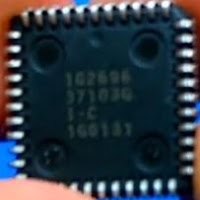PLCCA through-hole pin grid array package.